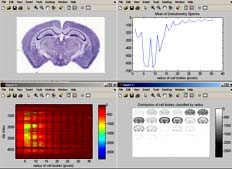## Downloads -> Desktop Applications -> BrainMaps AnalyzeBrainMaps Analyze is a powerful tool for applying image analysis routines to BrainMaps.org image data. There are currently two versions available: 1) a MATLAB version for Windows, Mac, and Linux, and 2) a command-line version for Mac and Linux (see below).

The image above is a screenshot of BrainMaps Analyze (Matlab version) performing granulometry analysis. The top left image is a thumbnail of the mouse brain section. The top right image shows the average (over tiles) granulometry spectrum, showing prominent peaks for different sized cells. The bottom left image contains a plot of cell size (x axis) vs tile number (y axis). The bottom right image is a plot of cell sizes, starting from radius equal to 1 pixel in the upper left, and incrementing by one in left to right, until it reaches radius of 36 pixels in the bottom right image. Note that the original image is over 22,000 pixels wide, and that the computation of granulometry was done on a per-image-tile basis, where each image tile is 256x256 pixels.

The BrainMaps Analyze code can be readily extended to include any types of image analysis.

### BrainMaps Analyze: MATLAB version for Windows, Mac, and Linux

The MATLAB program is provided below and may be run from within MATLAB. It is licensed under the GPL. The download also includes sample results, which may be plotted in Matlab by typing 'bmanalyzePlot'.

Usage:

There are four functions in the above download: 1) bmanalyze, 2) bmanalyzePlot, 3) bmanalyzeInfo, and 4) bmanalyzeGetPaths. These functions are described below.

```function h = bmanalyze( varargin )
% BMANALYZE Run analysis routines on BrainMaps.org high resolution tiled brain image data.
%   H = BMANALYZE(url) applies granulometry analysis on a per image
%   tile basis to brain image at url at the highest level of resolution.
%
%   H = BMANALYZE(url,function) applies 'function' on a per image
%   tile basis to brain image at url at the highest level of resolution.
%   Current functions are 'granulometry', 'mean', and 'var'.  It is
%   straightforward to add your own functions by editing the code below.
%
%   H = BMANALYZE(url,function,level) applies 'function' on a per image
%   tile basis to brain image at url at the given level of resolution.
%
%   Notes
%   -------
%   BMANALYZE may take several hours or even days to run.  If you want to see the type
%   of results BMANALYZE produces, try BMANALYZEPLOT.
%
%   To obtain image url's to use with BMANALYZE, use BMANALYZEGETPATHS, or
%   alternatively, find a brain dataset you
%   want to use at http://brainmaps.org, click on the 'Metadata' link, and
%   then click on 'Generate Paths' to generate a list of all url's for the
%   selected brain dataset.
%
%   Example
%   -------
%   Run Granulometry analysis on coronal mouse brain Nissl section.
%
%       bmanalyze(' http://brainmaps.org/HBP2/mus.musculus/cor/002-nissl/m08a/', 'granulometry');
%       bmanalyzePlot;
%

function h = bmanalyzePlot( )
% BMANALYZEPLOT Plot BMANALYZE results.
%   H = BMANALYZEPLOT() plots BMANALYZE results
%
%   Example
%   -------
%   Plot results from granulometry analysis on coronal mouse brain Nissl section.
%
%       bmanalyze('http://brainmaps.org/HBP2/mus.musculus/cor/002-nissl/m08a/', 'granulometry');
%       bmanalyzePlot;
%

function h = bmanalyzeInfo( varargin )
% BMANALYZEINFO(url) Returns image info of corresponding image 'url' from BrainMaps.org, including image
% height, width, number of tiles, and approximate computation time (assuming 1 sec per tile computation)
%
%   Notes
%   -------
%   To obtain image url's to use with BMANALYZE, use BMANALYZEGETPATHS, or
%   alternatively, find a brain dataset you
%   want to use at http://brainmaps.org , click on the 'Metadata' link, and
%   then click on 'Generate Paths' to generate a list of all url's for the
%   selected brain dataset.
%
%   Example
%   -------
%   Grab image info for brain image located at
%   http://brainmaps.org/HBP2/mus.musculus/cor/002-nissl/m08a/
%
%       bmanalyzeInfo(' http://brainmaps.org/HBP2/mus.musculus/cor/002-nissl/m08a/');
%

function h = bmanalyzeGetPaths( varargin )
% BMANALYZEGETPATHS Retrieves URLs for brain images from BrainMaps.org.
%   H = BMANALYZEGETPATHS(datid) retrieves URLs for BrainMaps.org for
%   given dataset (specified by datid).  Datid (which is a contraction of 'Data ID') is
%   an integer representing the unique ID of datasets. You can find out the
%   datid of a given dataset by hovering over the dataset's thumbnail at
%   brainmaps.org.  The value of datid ranges from 1 to however many
%   datasets are available (as of 06/13/2006, there are 43
%   datasets available).
%
%   Notes
%   -------
%   URLs retrieved by BMANALYZEGETPATHS may be used for BMANALYZE input.
%
%   An alternative way to obtain image url's for use with BMANALYZE is to find a brain dataset you
%   want to use at http://brainmaps.org, click on the 'Metadata' link, and
%   then click on 'Generate Paths' to generate a list of all url's for the
%   selected brain dataset.
%
%   Example
%   -------
%   Retrieve image URLs for dataset with datid=43
%
%       bmanalyzeGetPaths(43)
%
```

### BrainMaps Analyze: Command-line version and source code for Mac and Linux

The source code is provided below and may be compiled using GCC (GNU C Compiler). It is licensed under the GPL.

There are currently two image analysis routines that may be applied to BrainMaps image data: computations of 1) entropy, and 2) standard deviation of pixel values. Images at BrainMaps are composed of image tiles of size 256x256 pixels. BrainMaps Analyze performs image analysis on a per-image-tile basis and then maps the results of each computation to a corresponding pixel location, resulting in a lower-resolution map of the image analysis results (as shown in the image above). Users are free to create their own image analysis routines to be used with BrainMaps Analyze.

Usage:

summarize filter image-path

For example, at the command prompt, type the following:

summarize ppmentropy http://brainmaps.org/HBP2/m.mulatta/RH04/RH04-highres/0501

The above command will apply the filter "ppmentropy", which computes image entropy, to the image located at http://brainmaps.org/HBP2/m.mulatta/RH04/RH04-highres/0501